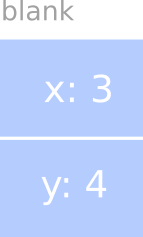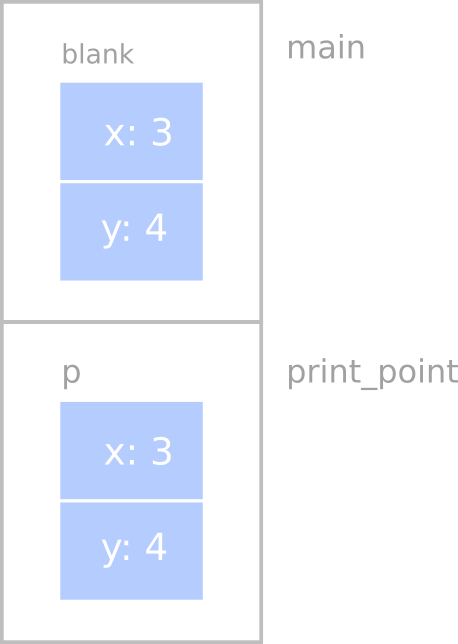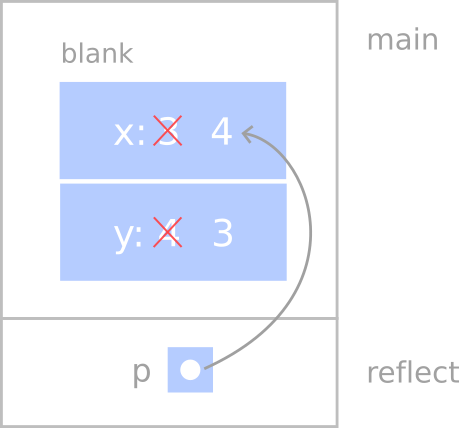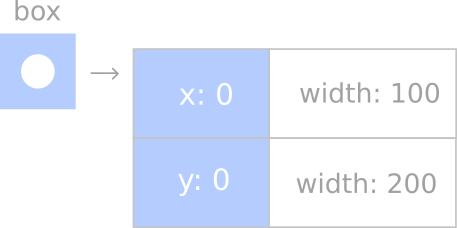# 8. Structures¶

## 8.1. Compound values¶

Most of the data types we have been working with represent a single value - an integer, a floating-point number, a boolean value. strings are different in the sense that they are made up of smaller pieces, the characters. Thus, strings are an example of a compound type.

Depending on what we are doing, we may want to treat a compound type as a single thing (or object), or we may want to access its parts (or instance variables). This ambiguity is useful.

It is also useful to be able to create your own compound values. C++ provides two mechanisms for doing that: structures and classes. We will start out with structures and get to classes in the Classes and invariants chapter.

## 8.2. Point objects¶

As a simple example of a compound structure, consider the concept of a mathematical point. At one level, a point is two numbers (coordinates) that we treat collectively as a single object. In mathematical notation, points are often written in parentheses, with a comma seperating the coordinates. For example, $$(0, 0)$$ indicates the origin, and $$(x, y)$$ indicates the point $$x$$ units to the right and $$y$$ units up from the origin.

A natural way to represent a point in C++ is with two doubless. The question, then, is how to group these two values into a compound object, or structure. The answer is a struct definition:

struct Point {
double x, y;
};


struct definitions appear outside of any function definition, usually at the beginning of the program (after the include statements).

This definition indicates that there are two elements in this structure, named x and y. These elements are called instance variables, for reasons we will explain a little later.

It is a common error to leave off the semi-colon at the end of a structure definition. It might seem odd to put a semi-colon after a curly brace, but you’ll get used to it.

Once you have defined the new structure, you can create variables with that type:

Point blank;
blank.x = 3.0;
blank.y = 3.0;


The first line is a conventional variable declaration: blank has type Point. The next two lines initialize the instance variables of the structure. The dot notation used here similar to the syntax for invoking a function on an object, as in the fruit.length() we saw in the Length section of the previous chapter. Of course, one difference is that function names are always followed by an argument list, even if it is empty.

The result of these arguments is shown in the following state diagram:As usual, the name of the variable blank appears outside the box and its value appears inside the box. In this case, the value is a compound object with two named instance variables.

## 8.3. Accessing instance variables¶

You can read the values of an instance variable using the same syntax we used to write them:

int x = blank.x;


The expression blank.x means “go to the object named blank and get the value of x.” In this case we assign that value to a local variable named x. Notice that there is no conflict between the local variable named x and the instance variable named x. The purpose of the dot notation is to identify which variable you are referring to unambigously.

You can use dot notation as part of any C++ expression, so the following are legal.

cout << "(" << blank.x << ", " << blank.y << ")" << endl;
double distance = blank.x * blank.x + blank.y * blank.y;


The first line outputs (3, 4); the second computes the value 25.

## 8.4. Operations on structures¶

Most of the operators we have been using on other types, like mathematical operators (+, %, etc.) and comparision operators (==, >, etc.), do not work on structures. Actually, it is possible to define the meaning of these operators for the new type, but we won’t do that in this book.

Note

Defining the meaning of built-in operators for new user defined types is called operator overloading. Not all programming languages support this feature, but C++ does.

On the other hand, the assignment operator does work for structures. It can be used in two ways: to initialize the instance variables of a structure or to copy the instance variables from one structure to another of the same type. An initialization looks like this:

Point blank = {3.0, 4.0};


The values in the curly braces get assigned to the instance variables of the structure one by one, in order. So in this case, x gets the first value and y gets the second.

Unfortunately, this syntax can only be used on an initialization, not in an assignment statement. So the following is illegal.

Point blank;
blank = {3.0, 4.0}     // WRONG!


You might wonder why this perfectly reasonable statement should be illegal, and there is no good answer. We’re sorry.

On the other hand, it is legal to assign one structure variable to another of the same type. For example:

Point p1 = {3.0, 4.0};
Point p2 = p1;
cout << "(" << p2.x << ", " << p2.y << ")" << endl;


The output of this program is (3, 4).

## 8.5. Structues as parameters¶

You can pass structures as parameters in the usual way. For example:

void print_point(Point p)
{
cout << "(" << p.x << ", " << p.y << ")" << endl;
}


print_point takes a point as an argument and outputs it in the standard format. If you call print_point(blank), it will output (3, 4).

As a second example, we can rewrite the distance function from the Program development section of chapter 5 so that it has two Point parameters instead of four doubles.

double distance(Point p1, Point p2)
{
double dx = p2.x - p1.x;
double dy = p2.y - p1.y;
return sqrt(dx*dx + dy*dy);
}


Remember to include the cmath library for sqrt.

## 8.6. Pass by value¶

When you pass a structure as an argument, remember that the argument and the parameter are not the same variable. Instead, there are two variables (one in the caller and one in the callee) that have the same value, at least initially. For example, when we call print_point, the stack diagram looks like this:If print_point happened to change one of the instance variables of p, it would have no effect on blank. Of course, there is no reason in this case for print_point to modify its parameter.

This kind of parameter passing is called pass by value because it is the value of the structure (or other type) that gets passed to the function.

## 8.7. Pass by reference¶

An alternative parameter passing mechanism that is available in C++ is called pass by reference. This mechanism makes it possible to pass a structure to a procedure and modify it.

For example, you can reflect a point around the 45-degree line by swapping the two coordinates. The most obvious (but incorrect) way to write a reflect function is something like this:

void reflect(Point p)    // WRONG!
{
double temp = p.x;
p.x = p.y;
p.y = temp;
}


But this won’t work, because the changes we make in reflect will have no effect on the caller.

Instead, we have to specify that we want to pass the parameter by reference. We do that by adding an ampersand (&) to the parameter declaration:

void reflect(Point& p)
{
double temp = p.x;
p.x = p.y;
p.y = temp;
}


Now we can call the function in the usual way:

print_point(blank);
reflect(blank);
print_point(blank);


The output of this code is as expected:

(3, 4)
(4, 3)


Here’s how we would draw a stack diagram for this code:The parameter p is a reference to the structure named blank. The usual representation for a reference is dot with an arrow that points to whatever the reference refers to.

The important thing to see in this diagram is that any changes that reflect makes to p will also change blank (since they are the same, not copies).

Passing structures by reference is more versatile than passing by value, because the callee can modify the structure. It is also faster, because the system does not have to copy the whole structure. On the other hand, is is less safe, since it is harder to keep track of what gets modified where. Nevertheless, in C++ programs, almost all structures are passed by reference almost all the time. We will follow that convention in this book.

## 8.8. Rectangles¶

Now let’s say that we want to create a structure to represent a rectangle. The question is, what information do we have to provide in order to specify a rectangle? To keep things simple let’s assume that the rectangle will be oriented vertically or horizontally, never at an angle.

There are a few possibilities: we could specify the center of the rectangle (two coordinates) and its size (width and height), or we could specify one of the corners and the size, or we could specify two opposing corners.

The most common choice in existing programs is to specify the upper left corner of the rectangle and the size. To do that in C++, we will define a structure that contains a Point and two doubles.

struct Rectangle {
Point corner;
double width, height;
};


Notice that one structure can contain another. In fact, this sort of thing is quite common. Of course, this means that in order to create a Rectangle, we have to create a Point first:

Point corner = {0.0, 0.0};
Rectangle box = {corner, 100.0, 200.0};


This code creates a new Point structure and initializes the instance variables. The figure shows the effect of this assignment.We can access the width and height in the usual way:

box.width += 50;
count << box.height << endl;


In order to access the instance variables of corner, we can use a temporary variable:

Point temp = box.corner;
double x = temp.x;


Alternatively, we can compose the two statements:

double x = box.corner.x;


It makes the most sense to read this statement from right to left: “Extract x from the corner of the box, and assign it to the local variable x.”

While on the subject of composition, we should point out that you can, in fact, create the Point and the Rectangle at the same time:

Rectangle box = {{0.0, 0.0}, 100.0, 200.0};


The innermost curly braces are the coordinates of the corner point; together they make up the first of the three values that go into the new Rectangle. This statement is an example of nested structure.

## 8.9. Structures as return types¶

You can write functions that return structures. For example, find_center takes a Rectangle as an argument and returns a Point that contains the coordinates of the center of the Rectangle:

Point find_center (Rectangle& box)
{
double x = box.corner.x + box.width/2;
double y = box.corner.y + box.height/2;
Point result = {x, y};
return result;
}


To call this function, we have to pass a box as an argument (notice that it is being passed by reference), and assign the return value to a Point variable:

Rectangle box = {{0, 0}, 100, 200};
Point center = find_center(box);
print_point(center);


The output of this program is (50, 100).

## 8.10. Passing other types by reference¶

It’s not just structures that can be passed by reference. All the other types we’ve seen can too. For example, to swap two integers, we could write something like:

void swap(int& x, int& y)
{
int temp = x;
x = y;
y = temp;
}


We would call this function in the usual way:

int i = 7;
int j = 9;
swap(i, j);
cout << i << j << endl;


The output of this program is 97. Draw a stack diagram for this program to convince yourself this is true. If the parameters x and y were declared as regular parameters (without the &s), swap would not work. It would modify x and y and have no effect on i and j.

When people start passing things like integers by reference, they often try to use an expression as a reference argument. For example:

int i = 7;
int j = 9;
swap(i, j+1);     // WRONG!


This is not legal because the expression j+1 is not a variable - it does not occupy a location that the reference can refer to. It is a little tricky to figure out exactly what kinds of expressions can be passed by reference. For now a good rule of thumb is that reference arguments have to be variables.

## 8.11. Glossary¶

structure

A collection of data grouped together and treated as a single object.

instance variable

One of the named pieces of data that make up a structure.

reference

A value that indicates or refers to a variable or structure. In a state diagram, a reference appears as an arrow.

pass by value

A method of parameter passing in which the value provided as an argument is copied into the corresponding parameter, but the parameter and the argument occupy distinct locations.

pass by reference

A method of parameter passing in which the parameter is a reference to the argument variable. Changes to the parameter also effect the argument variable.

## 8.12. Exercises¶

1. Write function named lowerRight that takes a Rectangle as an argument and returns a Point with the location of the lower right hand corner.

2. Write a function named findArea that takes a Rectangle as an argument and returns the area of the rectangle.